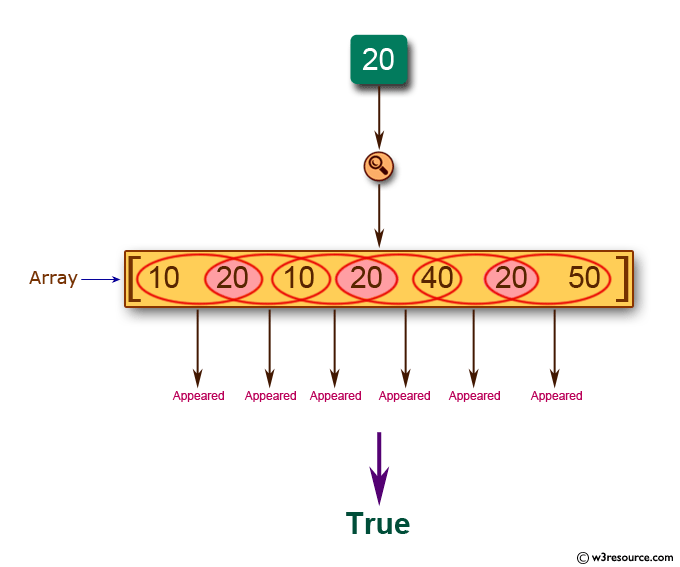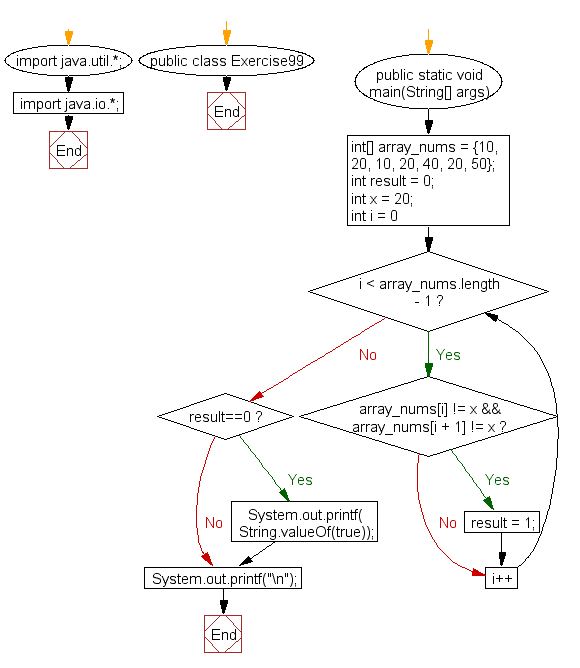﻿ Java: If a number occurs in every paired element in an array# Java Exercises: Check if a specified number appears in every pair of adjacent element of a given array of integers

## Java Basic: Exercise-99 with Solution

Write a Java program to check if a specified number appears in every pair of adjacent element of a given array of integers.

Pictorial Presentation:Sample Solution:

Java Code:

``````import java.util.*;
import java.io.*;
public class Exercise99 {
public static void main(String[] args)
{
int[] array_nums = {10, 20, 10, 20, 40, 20, 50};
int result = 0;
int x = 20;
for(int i = 0; i < array_nums.length - 1; i++) {
if(array_nums[i] != x && array_nums[i + 1] != x)
{
result = 1;
}
}
if (result==0)
{
System.out.printf( String.valueOf(true));
}
else
{
System.out.printf( String.valueOf(false));
}
}
}
```
```

Sample Output:

```true
```

Flowchart:Java Code Editor:

What is the difficulty level of this exercise?

Test your Programming skills with w3resource's quiz.

﻿

## Java: Tips of the Day

countOccurrences

Counts the occurrences of a value in an array.

Use Arrays.stream().filter().count() to count total number of values that equals the specified value.

```public static long countOccurrences(int[] numbers, int value) {
return Arrays.stream(numbers)
.filter(number -> number == value)
.count();
}
```

Ref: https://bit.ly/3kCAgLb